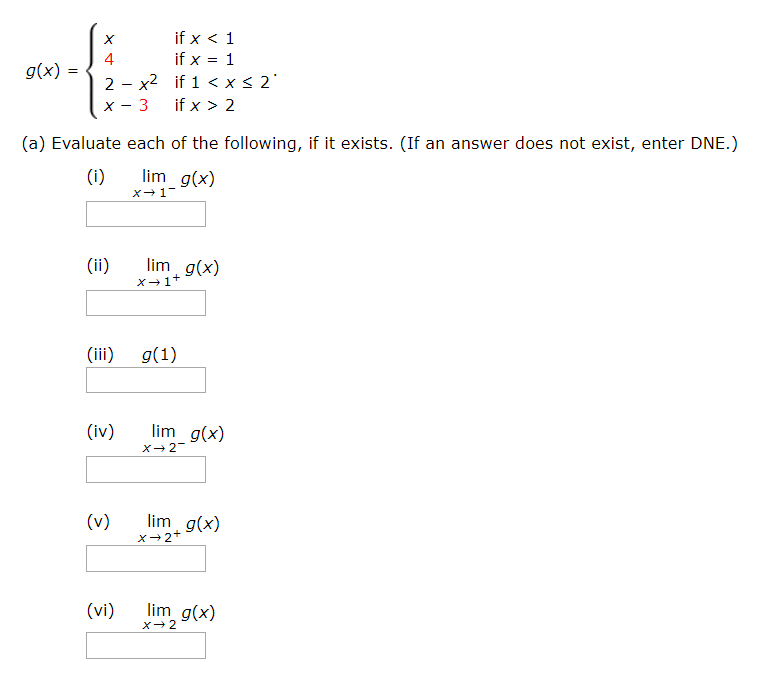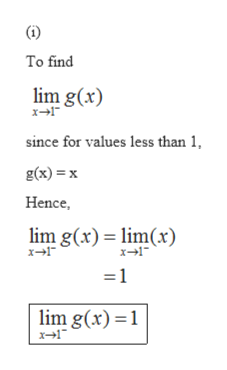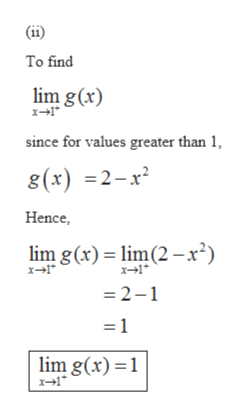# if x < 1if x = 14g(x):2 - x2 if 1 < x< 2'if x > 2(a) Evaluate each of the following, if it exists. (If an answer does not exist, enter DNE.)lim g(x)(i)lim g(x)(ii)(iii)g(1)lim g(x)(iv)lim g(x)(v)lim g(x)(vi)

Question
1 viewshelp_outlineImage Transcriptioncloseif x < 1 if x = 1 4 g(x): 2 - x2 if 1 < x< 2' if x > 2 (a) Evaluate each of the following, if it exists. (If an answer does not exist, enter DNE.) lim g(x) (i) lim g(x) (ii) (iii) g(1) lim g(x) (iv) lim g(x) (v) lim g(x) (vi) fullscreen
check_circle

Step 1

Given function is

Step 2

Evaluating, we gethelp_outlineImage Transcriptionclose(i) To find lim g(x) since for values less than 1, g(x) = x Hence, lim g(x) = lim(x) =1 lim g(x) =1 fullscreen
Step 3help_outlineImage Transcriptionclose(ii) To find lim g (x) x→1* since for values greater than 1, 8(x) =2-x² Hence, lim g(x) = lim(2 -x²) x- = 2-1 lim g(x)=1 fullscreen

### Want to see the full answer?

See Solution

#### Want to see this answer and more?

Solutions are written by subject experts who are available 24/7. Questions are typically answered within 1 hour.*

See Solution
*Response times may vary by subject and question.
Tagged in

### Functions